## First Example done together: An Excel budget, expenses sheet template

I guess everyone likes to know where he puts his money and how it is spent. So starting with a budget or expense sheet would be a great starter.

We will see how to create the budget sheet, the first table, enter the various items you need to make a budget, add charts that show us visualy if we are broke or not....

This is the result we are going to reach. One table where you enter the data and another chart where you can easily see what you spent.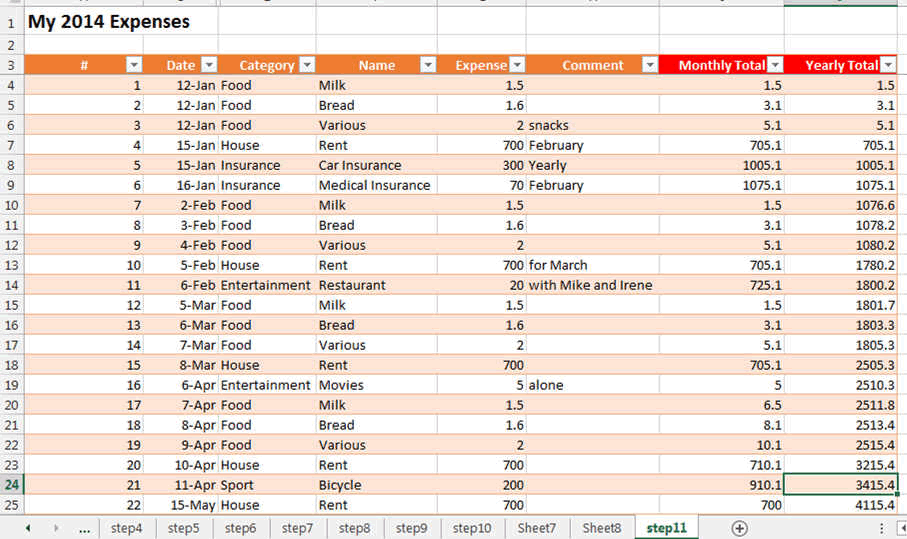This is the Excel chart that displays your expenses and budget.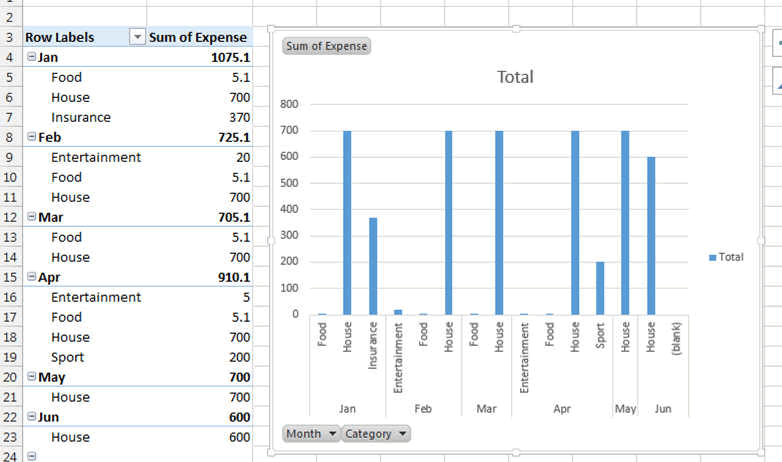Of course the colours, the styles are can be changed to your likings.

### Let's Start

Lets define what we need to track our expenses. We need to enter a date, the item name, the expense and a comment maybe.

Excel has to calculate by itself, the monthly total, the yearly total and then display it.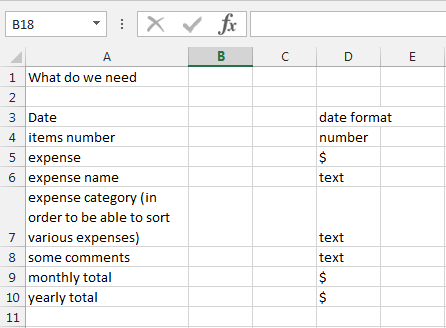In the new blank worksheet we are going to enter the headers of our columns.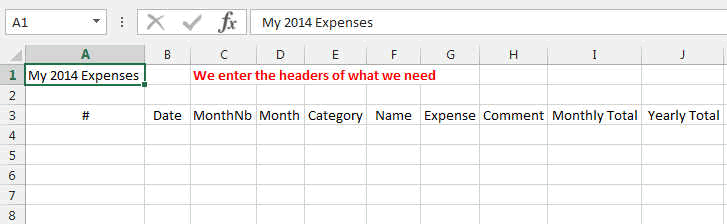We can also enter some dummy data, or you can enter some real data if you want.Lets create the table now. We are going to plan for a table with 2000 entries. But you can change this.

So select all the cells that contain the titles. Then press CTRL-T (or the Insert TABLE button in the INSERT Ribbon).

You can also press on the Format as Table button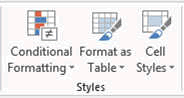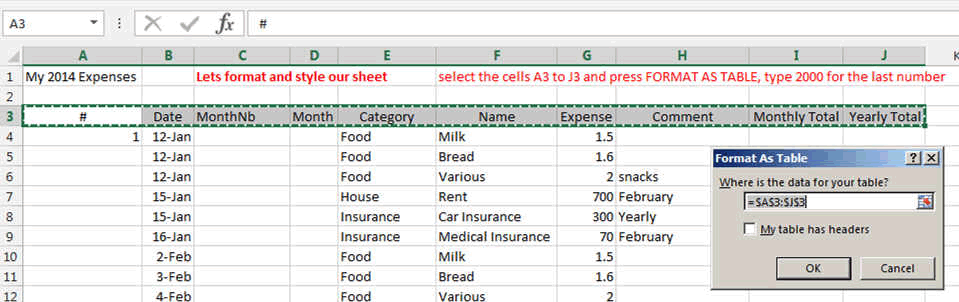In this window, change the number behind the \$ sign to 2000. Select MY TABLE HAS HEADERS and press OK.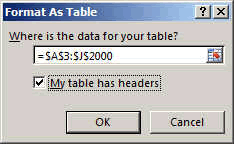A new table should be created now looking like this (may the color scheme is different but you can change this in the table Design menu)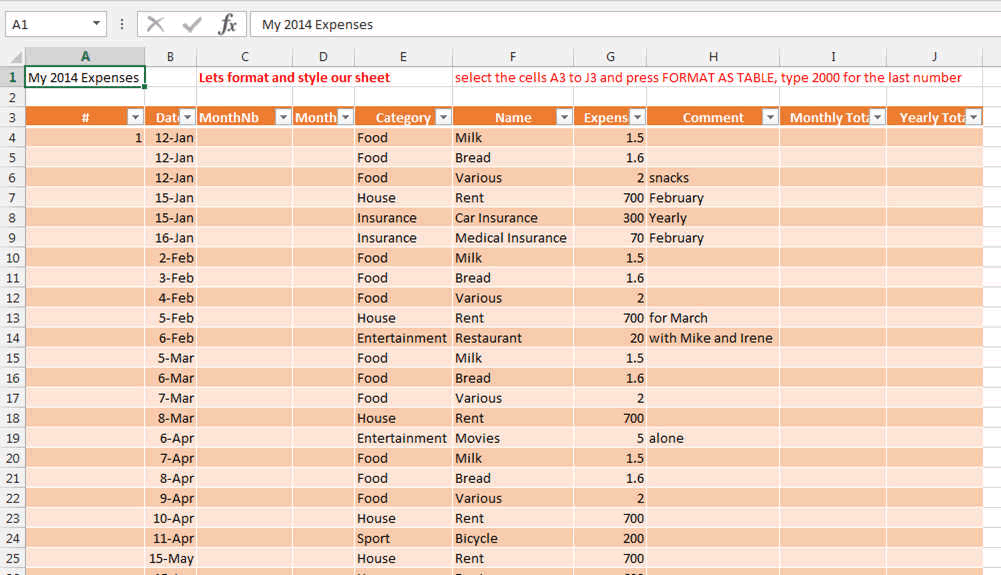Now we have to enter some formulas where the calculation will be done.

Type what you see on the screen.

See how the date is transformed into a real number... the number 1 represents the 1 January 1900

You can see the formulas by clicking in the Ribbon FORMULAS the options "show formulas"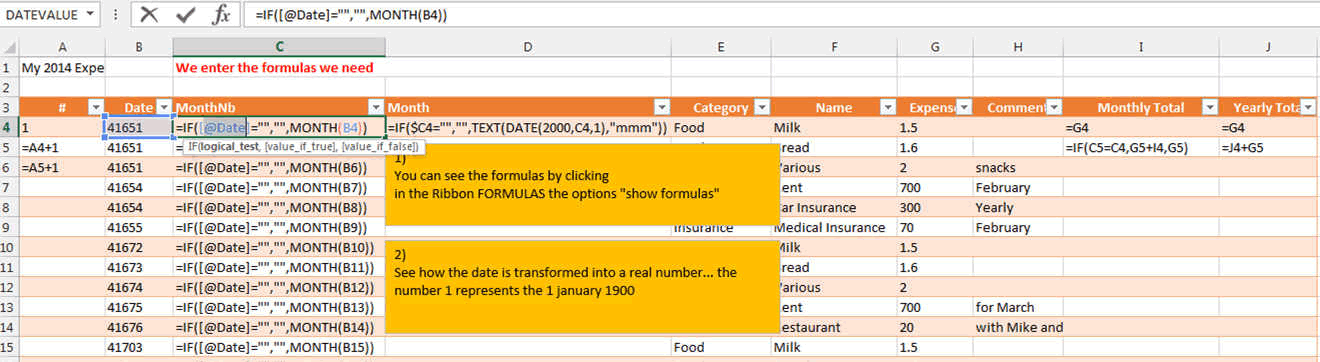in C4 : =IF([@Date]="","",MONTH(B4))

From the date you have entered, this takes only the month (1-12)

in D4: =IF(\$C4="","",TEXT(DATE(2000,C4,1),"mmm"))

This transforms the month (1-12) into Jan to Dec

in I4: =G4

This is to initialize the first row of the monthly expenses

in I5: =IF(C5=C4,G5+I4,G5)

This adds to the monthly expenses the new expense, but only if the month is the same (C5 = C4)

in J4: =I4

This is to initialize the first row of the yearly expenses

in J5: =J4+G5

This sums the yearly expenses.

SO SIMPLE; isn't it?

Lets go the the next page.